Method 2

The second method for solving exponential equations involves using logarithms. For example, when trying to solve 3x = 11, 3 and 11 cannot be written as like bases. The log of both sides will need to be determined.

Example 1 Solve the equation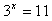Step 1. Since there is no common base, apply logarithms.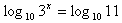In this example, common logs were used but any base can be used.

Step 2. Use the Power to a Power Property.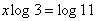Step 3. Solve for x.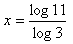*Note: If starting with a different base, the Change of Base Property will create this same equation.

Step 4. Use a calculator to find the answer.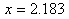Step 5. Make sure to check the answer: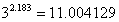*Note: Since the value of x was rounded to three decimal places it creates a round-off error (a slightly inaccurate answer when checked).